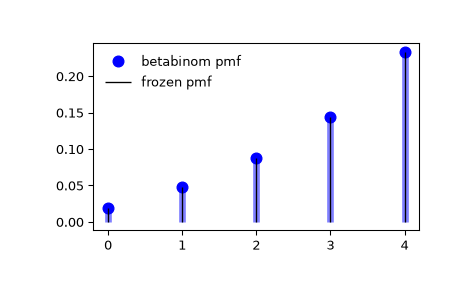# scipy.stats.betabinom¶

scipy.stats.betabinom(*args, **kwds) = <scipy.stats._discrete_distns.betabinom_gen object>[source]

A beta-binomial discrete random variable.

As an instance of the rv_discrete class, betabinom object inherits from it a collection of generic methods (see below for the full list), and completes them with details specific for this particular distribution.

Notes

The beta-binomial distribution is a binomial distribution with a probability of success p that follows a beta distribution.

The probability mass function for betabinom is:

$f(k) = \binom{n}{k} \frac{B(k + a, n - k + b)}{B(a, b)}$

for k in {0, 1,..., n}, $$n \geq 0$$, $$a > 0$$, $$b > 0$$, where $$B(a, b)$$ is the beta function.

betabinom takes $$n$$, $$a$$, and $$b$$ as shape parameters.

References

1

https://en.wikipedia.org/wiki/Beta-binomial_distribution

The probability mass function above is defined in the “standardized” form. To shift distribution use the loc parameter. Specifically, betabinom.pmf(k, n, a, b, loc) is identically equivalent to betabinom.pmf(k - loc, n, a, b).

New in version 1.4.0.

Examples

>>> from scipy.stats import betabinom
>>> import matplotlib.pyplot as plt
>>> fig, ax = plt.subplots(1, 1)


Calculate a few first moments:

>>> n, a, b = 5, 2.3, 0.63
>>> mean, var, skew, kurt = betabinom.stats(n, a, b, moments='mvsk')


Display the probability mass function (pmf):

>>> x = np.arange(betabinom.ppf(0.01, n, a, b),
...               betabinom.ppf(0.99, n, a, b))
>>> ax.plot(x, betabinom.pmf(x, n, a, b), 'bo', ms=8, label='betabinom pmf')
>>> ax.vlines(x, 0, betabinom.pmf(x, n, a, b), colors='b', lw=5, alpha=0.5)


Alternatively, the distribution object can be called (as a function) to fix the shape and location. This returns a “frozen” RV object holding the given parameters fixed.

Freeze the distribution and display the frozen pmf:

>>> rv = betabinom(n, a, b)
>>> ax.vlines(x, 0, rv.pmf(x), colors='k', linestyles='-', lw=1,
...         label='frozen pmf')
>>> ax.legend(loc='best', frameon=False)
>>> plt.show()Check accuracy of cdf and ppf:

>>> prob = betabinom.cdf(x, n, a, b)
>>> np.allclose(x, betabinom.ppf(prob, n, a, b))
True


Generate random numbers:

>>> r = betabinom.rvs(n, a, b, size=1000)


Methods

 rvs(n, a, b, loc=0, size=1, random_state=None) Random variates. pmf(k, n, a, b, loc=0) Probability mass function. logpmf(k, n, a, b, loc=0) Log of the probability mass function. cdf(k, n, a, b, loc=0) Cumulative distribution function. logcdf(k, n, a, b, loc=0) Log of the cumulative distribution function. sf(k, n, a, b, loc=0) Survival function (also defined as 1 - cdf, but sf is sometimes more accurate). logsf(k, n, a, b, loc=0) Log of the survival function. ppf(q, n, a, b, loc=0) Percent point function (inverse of cdf — percentiles). isf(q, n, a, b, loc=0) Inverse survival function (inverse of sf). stats(n, a, b, loc=0, moments=’mv’) Mean(‘m’), variance(‘v’), skew(‘s’), and/or kurtosis(‘k’). entropy(n, a, b, loc=0) (Differential) entropy of the RV. expect(func, args=(n, a, b), loc=0, lb=None, ub=None, conditional=False) Expected value of a function (of one argument) with respect to the distribution. median(n, a, b, loc=0) Median of the distribution. mean(n, a, b, loc=0) Mean of the distribution. var(n, a, b, loc=0) Variance of the distribution. std(n, a, b, loc=0) Standard deviation of the distribution. interval(alpha, n, a, b, loc=0) Endpoints of the range that contains alpha percent of the distribution

#### Previous topic

scipy.stats.bernoulli

#### Next topic

scipy.stats.binom